# 4.8: Covalent Bonding and Formula Writing

•• Contributed by Elizabeth Gordon
• Lecturer (Chemistry) at Furman University

Learning Objectives

• To know what types of elements bond to form covalent compounds.
• To appreciate how atoms share their valence electrons in covalent bonding.
• Memorize numerical prefixes used in covalent nomenclature
• Understand that covalent compound subscripts are never reduced.

## Electron Sharing

Previously, we discussed ionic bonding where electrons can be transferred from one atom to another so that both atoms have an energy-stable outer electron shell. Because most filled electron shells have eight electrons in them, chemists called this tendency the octet rule. However, there is another way an atom can achieve a full valence shell: atoms can share electrons. This type of bonding would be a covalent bond. Two combinations of atoms can produce this type of bonding: nonmetal/nonmetal or metalloid/nonmetal. In this class, we will not discuss the option of metallic bonding which is a form of covalent bonding.Figure $$\PageIndex{1}$$: Sharing is caring, especially for atoms that participate in covalent bonding. Image used with permission (CC BY-2.0; Carlos Maya).

This concept can be illustrated by using two hydrogen atoms, each of which has a single electron in its valence shell. (For small atoms such as hydrogen atoms, the valence shell will be the first shell, which holds only two electrons.) We can represent the two individual hydrogen atoms as follows:In contrast, when two hydrogen atoms get close enough together to share their electrons, they can be represented as follows: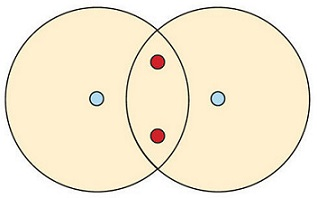By sharing their valence electrons, both hydrogen atoms now have two electrons in their respective valence shells. Because each valence shell is now filled, this arrangement is more stable than when the two atoms are separate. The sharing of electrons between atoms is called a covalent bond, and the two electrons that join atoms in a covalent bond are called a bonding pair of electrons. A discrete group of atoms connected by covalent bonds is called a molecule—the smallest part of a compound that retains the chemical identity of that compound.

Chemists frequently use Lewis diagrams to represent covalent bonding in molecular substances. For example, the Lewis diagrams of two separate hydrogen atoms are as follows: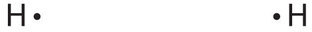The Lewis diagram of two hydrogen atoms sharing electrons looks like this:This depiction of molecules is simplified further by using a dash to represent a covalent bond. The hydrogen molecule is then represented as follows:Remember that the dash, also referred to as a single bond, represents a pair of electrons.

The bond in a hydrogen molecule, measured as the distance between the two nuclei, is about 7.4 × 10−11 m, or 74 picometers (pm; 1 pm = 1 × 10−12 m). This particular bond length represents a balance between several forces: the attractions between oppositely charged electrons and nuclei, the repulsion between two negatively charged electrons, and the repulsion between two positively charged nuclei. If the nuclei were closer together, they would repel each other more strongly; if the nuclei were farther apart, there would be less attraction between the positive and negative particles.

Fluorine is another element whose atoms bond together in pairs to form diatomic (two-atom) molecules. Two separate fluorine atoms have the following electron dot diagrams: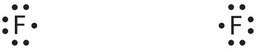Each fluorine atom contributes one valence electron, making a single bond and giving each atom a complete valence shell, which fulfills the octet rule:The circles show that each fluorine atom has eight electrons around it. As with hydrogen, we can represent the fluorine molecule with a dash in place of the bonding electrons: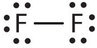Each fluorine atom has six electrons, or three pairs of electrons, that are not participating in the covalent bond. Rather than being shared, they are considered to belong to a single atom. These are called nonbonding pairs (or lone pairs) of electrons.

## Covalent Bonds between Different Atoms

Now that we have looked at electron sharing between atoms of the same element, let us look at covalent bond formation between atoms of different elements. Consider a molecule composed of one hydrogen atom and one fluorine atom:Each atom needs one additional electron to complete its valence shell. By each contributing one electron, they make the following molecule:In this molecule, the hydrogen atom does not have nonbonding electrons, while the fluorine atom has six nonbonding electrons (three lone electron pairs). The circles show how the valence electron shells are filled for both atoms.

Example $$\PageIndex{1}$$

Draw the Lewis diagram for each compound.

1. a molecule composed of two chlorine atoms
2. a molecule composed of a hydrogen atom and a bromine atom

SOLUTION

1. Chlorine has the same valence shell electron configuration as fluorine, so the Lewis diagram for a molecule composed of two chlorine atoms is similar to the one for fluorine:2. Bromine has the same valence shell electron configuration as fluorine, so the Lewis diagram for a molecule composed of a hydrogen atom and a bromine atom is similar to that for hydrogen and fluorine:Exercise $$\PageIndex{1}$$

Draw the Lewis diagram for each compound (show both formats).

1. a molecule composed of one chlorine atom and one fluorine atom
2. a molecule composed of one hydrogen atom and one iodine atomChlorine monofluoride is a volatile interhalogen compound.$$\ce{HI}$$ is used in organic and inorganic synthesis as one of the primary sources of iodine.

Larger molecules are constructed in a similar fashion, with some atoms participating in more than one covalent bond. For example, water, with two hydrogen atoms and one oxygen atom can be represented as follows:and methane (CH4), with one carbon atom and four hydrogen atoms, can also be represented as follows:Figure $$\PageIndex{1}$$ shows the number of covalent bonds various atoms typically form.

Rules for drawing Covalent Lewis Structures

These apply to either nonmetal/nonmetal or metalloid/nonmetal combinations.

1. Tally up all valence electrons that are to be used. Remember valence electrons are the roman numerals above the element.
2. Determine which atom is to be central. Carbon is always central and Hydrogen is a terminal atom. If these atoms are not present, then go with the atom you have least of in the formula.
3. Connect each atom to the central atom using single bonds first.
4. For simple molecules or ions (only have two atoms), try single bonds, then double, and finally triple to achieve octets around each atom (except for B, Be, and H)
5. For more complex molecules or ions (3 or more atoms), try one multiple bond at a time if single bonds achieve octets.
6. My series for molecules/ions that need more than single bonds can be seen below (keeping in mind H, B, and Be only have single bonds)
• Single/single ($$\ce{-}$$, $$\ce{-}$$) ,
• then single/double ($$\ce{-}$$, $$\ce{=}$$),
• then double/double ($$\ce{=}$$, $$\ce{=}$$),
• and if that does not work try triple/single ($$\ce{#}$$, $$\ce{-}$$)
1. In our class, we do not use multiple bonds for halogens.

## Double and Triple Bonds

In covalent bonding, using double (four total electrons) or triple (six total electrons) is an option in order to achieve a stable octet. If a single bond does not work, then you may incorporate a multiple bond. Please note the rules above on which atoms will never achieve octets (hydrogen and boron). Although it is mathematically feasible, halogens will not use multiple bonds to become stable either.

Let's look at a few molecules that utilize multiple bonds:Atoms that could employ multiple bonds would be oxygen, nitrogen, sulfur, phosphorus, and carbon. If an octet can be achieved with a single bond, do not incorporate multiple bonds into the structure.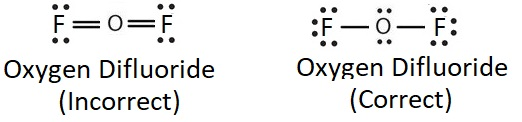Multiple bonds involve more that one type of connection. For this reason, it is more difficult to break or cleave a multiple bond than it would be a single bond or a bond of a lower order (when comparing the same connected atoms). For example, the triple bond found in carbon monoxide is harder to break than one of the double bonds found in carbon dioxide. When reading about smog production or ozone depletion, consider the bond strengths of the chemicals involved in this environmental problems.

Example $$\PageIndex{1}$$

Before drawing the structures below, access the Furman University CHM101 moodle documents, Here, you will see a lightboard video of your instructor drawing Lewis structures for a variety of covalent compounds. Then, try to construct structures of the other molecules in this example.

1. O3 (ozone)
2. N2 (nitrogen)
3. SO3 (sulfur trioxide)
4. CCl4 (carbon tetrachloride)

Solutions## Formula Writing for Covalent Compounds

Covalent compounds use prefixes to indicate the numbers of atoms in their formulas. In order to write correct formulas, you will need to memorize Table $$\PageIndex{1}$$ below.

Table $$\PageIndex{1}$$: Prefix to occurrence correlations.
Prefix name Number of Atoms
Mono 1
Di (not bi) 2
Tri 3
Penta 5
Hexa 6
Hepta (not septa) 7
Octa 8
Nona 9
Deca 10

Rules for Writing Covalent formulas

1. There are no charges involved with covalent compounds.
2. Interpret the prefixes to represent the number of atoms or the subscript.
3. Do not cross or reduce subscripts (unlike ionic compounds).
4. The formula should reflect the name of the compound.

Example $$\PageIndex{1}$$

Write formulas for the following covalent compounds:

1. dinitrogen pentoxide
2. boron trihydride
3. sulfur hexafluoride
4. carbon tetrachloride
5. silicon dioxide
6. dihydrogen dioxide

N2O5

BH3

SF6